# 1. 控制变量

## 0x2：控制变量思想在机器学习中的应用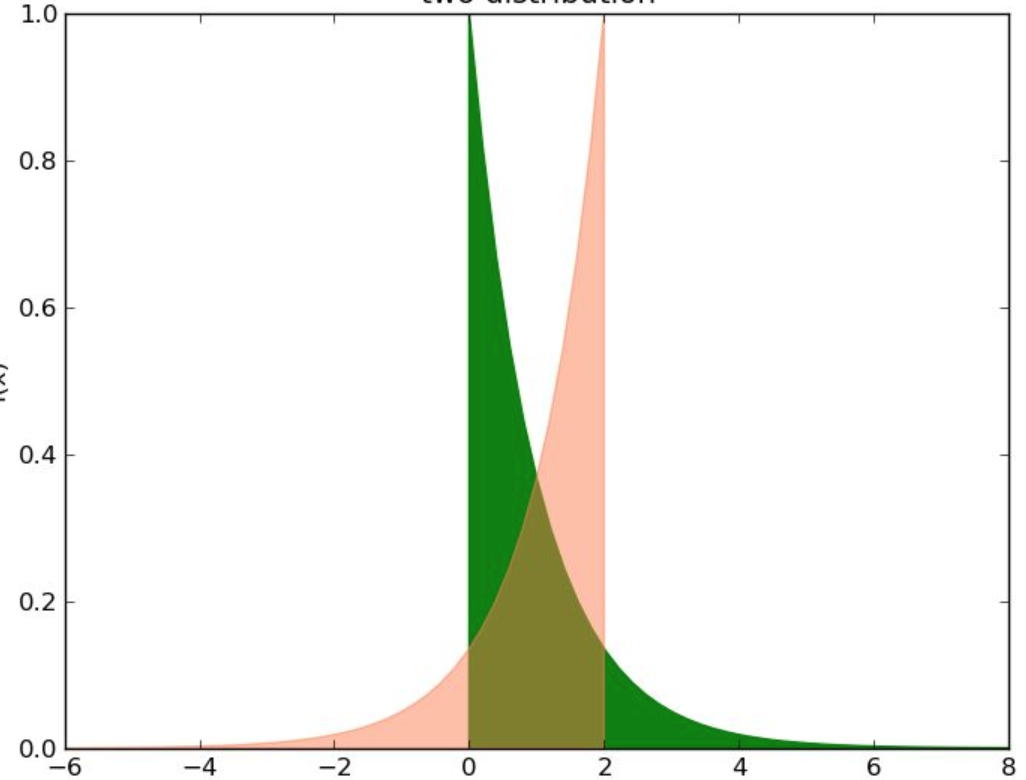## 0x3：控制变量思想在贝叶斯统计推断中的作用

https://wenku.baidu.com/view/afe92b8fb04e852458fb770bf78a6529647d35c7.html

# 2. A/B测试简介

## 0x1：A/B测试基本思想

A/B测试背后的基本思想是：假如有一个理想的平行宇宙用于对照，该宇宙的人与我们这里的人是完全一样的（除控制变量外，其他变量都相同），那么此时如果给某一边的人以某种特殊的待遇（调整控制变量），那么结果所导致的变化一定会被归咎于这一特殊待遇。

## 0x2：A/B测试的最终目的

A/B测试的最终目的是比较A/B的后验分布的差异性，使用的方法有很多种，例如t-test。

## 0x3：A/B测试的优势

1. 可解释的概率值。在贝叶斯分布中，我们可以直接回答诸如”我们出错的概率是多少“之类的问题，这在频率派的方法中通常难以回答；
2. 很容易应用损失函数；

https://baike.baidu.com/item/AB%E6%B5%8B%E8%AF%95/9231223?fr=aladdin

# 3. 二项分布问题场景的A/B测试 - 一个网站转化率测试案例

visitors_to_A = 1300；
visitors_to_B = 1300；
conversions_from_A = 120；
conversions_from_B = 125；

## 0x1：选择先验分布

Beta分布的共轭特性是的我们不需要进行MCMC，可以直接得到后验概率分布。

## 0x2：计算后验分布

# -*- coding: utf-8 -*-

import numpy as np
import matplotlib.pyplot as plt
import scipy.stats as stats
import pymc as pm
from scipy.stats import beta

visitors_to_A = 1300
visitors_to_B = 1300
conversions_from_A = 120
conversions_from_B = 125

alpha_prior = 1
beta_prior = 1

posterior_A = beta(alpha_prior + conversions_from_A,
beta_prior + visitors_to_A - conversions_from_A)

posterior_B = beta(alpha_prior + conversions_from_B,
beta_prior + visitors_to_B - conversions_from_B)

plt.show()

## 0x3：比较后验分布的大小

### 1. MCMC后验采样法

# -*- coding: utf-8 -*-

import numpy as np
import matplotlib.pyplot as plt
import scipy.stats as stats
import pymc as pm
from scipy.stats import beta

visitors_to_A = 1300
visitors_to_B = 1300
conversions_from_A = 120
conversions_from_B = 125

alpha_prior = 1
beta_prior = 1

posterior_A = beta(alpha_prior + conversions_from_A,
beta_prior + visitors_to_A - conversions_from_A)

posterior_B = beta(alpha_prior + conversions_from_B,
beta_prior + visitors_to_B - conversions_from_B)

samples = 2000
samples_posterior_A = posterior_A.rvs(samples)
samples_posterior_B = posterior_B.rvs(samples)

print(samples_posterior_A > samples_posterior_B).mean()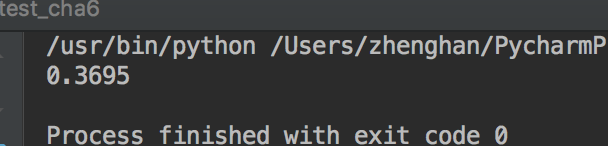# -*- coding: utf-8 -*-

import numpy as np
import matplotlib.pyplot as plt
import scipy.stats as stats
import pymc as pm
from scipy.stats import beta
from IPython.core.pylabtools import figsize

visitors_to_A = 1300
visitors_to_B = 1300
conversions_from_A = 120
conversions_from_B = 125

alpha_prior = 1
beta_prior = 1

posterior_A = beta(alpha_prior + conversions_from_A,
beta_prior + visitors_to_A - conversions_from_A)

posterior_B = beta(alpha_prior + conversions_from_B,
beta_prior + visitors_to_B - conversions_from_B)

samples = 2000
samples_posterior_A = posterior_A.rvs(samples)
samples_posterior_B = posterior_B.rvs(samples)

print(samples_posterior_A > samples_posterior_B).mean()

figsize(12.5, 4)

plt.rcParams['savefig.dpi'] = 300
plt.rcParams['figure.dpi'] = 300
x = np.linspace(0, 1, 500)
plt.plot(x, posterior_A.pdf(x), label='posterior of A')
plt.plot(x, posterior_B.pdf(x), label='posterior of B')
plt.xlim(0.05, 0.15)
plt.xlabel('value')
plt.ylabel('density')
plt.show()# 4.  多项分布下的A/B测试

## 0x1：收入期望的分析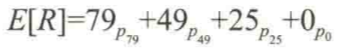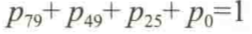## 0x2：选择概率分布模型

Beta分布有一个推广，叫做 Dirichlet（狄利克雷）分布。它返回一个和为 1 的正数数组。数组的长度由一个输入向量的长度决定，这一输入向量的值类似于先验的参数。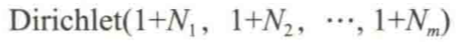## 0x3：计算后验分布

# -*- coding: utf-8 -*-

import numpy as np
import matplotlib.pyplot as plt
import scipy.stats as stats
import pymc as pm
from numpy.random import dirichlet
from IPython.core.pylabtools import figsize

N = 1000
N_79 = 10
N_49 = 46
N_25 = 80
N_0 = N - (N_79 + N_49 + N_25)

observations = np.array([N_79, N_49, N_25, N_0])

prior_parameters = np.array([1, 1, 1, 1])
posterior_samples = dirichlet(prior_parameters+observations, size=10000)

print "Two random samples from the posterior: "
print posterior_samples
print posterior_samples

for i, label in enumerate(['p_79', 'p_49', 'p_25', 'p_0']):
ax = plt.hist(posterior_samples[:, i], bins=50, label=label, histtype='stepfilled')

plt.xlabel('value')
plt.ylabel('density')
plt.show()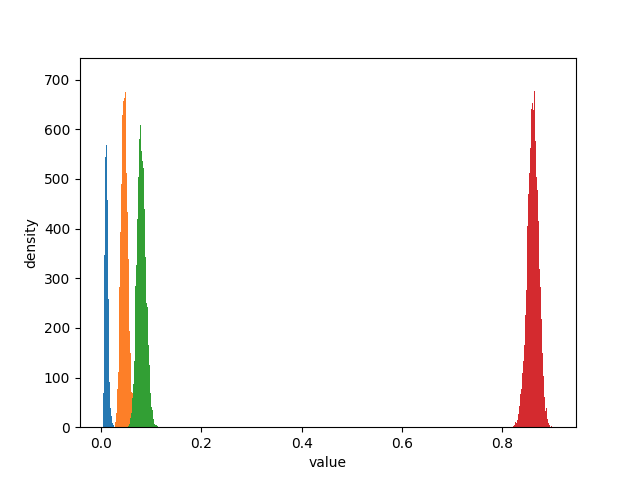## 0x4：计算总收入期望的后验分布

# -*- coding: utf-8 -*-

import numpy as np
import matplotlib.pyplot as plt
import scipy.stats as stats
import pymc as pm
from numpy.random import dirichlet
from IPython.core.pylabtools import figsize

N = 1000
N_79 = 10
N_49 = 46
N_25 = 80
N_0 = N - (N_79 + N_49 + N_25)

observations = np.array([N_79, N_49, N_25, N_0])

prior_parameters = np.array([1, 1, 1, 1])
posterior_samples = dirichlet(prior_parameters+observations, size=10000)

def expected_revenue(P):
return 79 * P[:, 0] + 49 * P[:, 1] + 25 * P[:, 2] + 0 * P[:, 3]

posterior_expected_revenue = expected_revenue(posterior_samples)

plt.hist(posterior_expected_revenue, histtype='stepfilled', label='expected revenue', bins=50)
plt.xlabel('value')
plt.ylabel('density')
plt.show()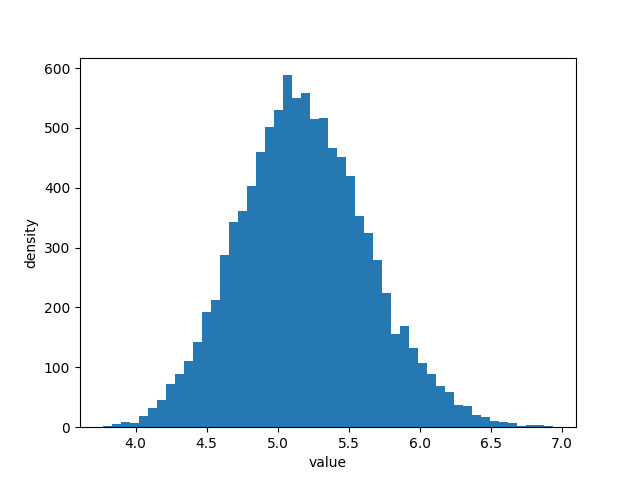1. 收入的期望值有很大可能在 $4 和$6 之间，不大可能在这个范围之外

## 0x5：对两个不同的页面进行分析 - 延伸到A/B测试

### 1. 计算后验分布

# -*- coding: utf-8 -*-

import numpy as np
import matplotlib.pyplot as plt
import scipy.stats as stats
import pymc as pm
from numpy.random import dirichlet
from IPython.core.pylabtools import figsize

N_A = 1000
N_A_79 = 10
N_A_49 = 46
N_A_25 = 80
N_A_0 = N_A - (N_A_79 + N_A_49 + N_A_25)
observations_A = np.array([N_A_79, N_A_49, N_A_25, N_A_0])

N_B = 2000
N_B_79 = 45
N_B_49 = 84
N_B_25 = 200
N_B_0 = N_B - (N_B_79 + N_B_49 + N_B_25)
observations_B = np.array([N_B_79, N_B_49, N_B_25, N_B_0])

prior_parameters = np.array([1, 1, 1, 1])

posterior_samples_A = dirichlet(prior_parameters+observations_A, size=10000)
posterior_samples_B = dirichlet(prior_parameters+observations_B, size=10000)

def expected_revenue(P):
return 79 * P[:, 0] + 49 * P[:, 1] + 25 * P[:, 2] + 0 * P[:, 3]

posterior_expected_revenue_A = expected_revenue(posterior_samples_A)
posterior_expected_revenue_B = expected_revenue(posterior_samples_B)

plt.hist(posterior_expected_revenue_A, histtype='stepfilled', label='expected revenue A', bins=50)
plt.hist(posterior_expected_revenue_B, histtype='stepfilled', label='expected revenue B', bins=50, alpha=0.8)

plt.xlabel('value')
plt.ylabel('density')
plt.show()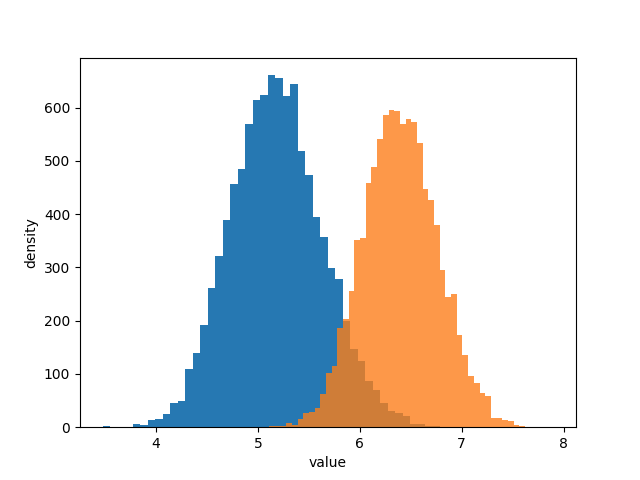### 2. 从后验分布概率图中我们得到了什么初步的推断信息

1. 两个后验的距离比较远，说明两种页面的表现有很多差别，但这里只是一个感性的认知，具体差别多少不知道；

## 0x6：A/B两组后验的增幅的估计

1. 连续值问题是要衡量结果到底好多少，这是一定范围内的连续值（软分界）；
2. 二值问题是要判断谁更好，只有两种可能（硬分界）；

### 1. 用后验分布的均值计算相对增幅合理吗？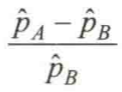### 2. 计算后验分布的概率密度增幅

# -*- coding: utf-8 -*-

import numpy as np
import matplotlib.pyplot as plt
import scipy.stats as stats
import pymc as pm
from scipy.stats import beta
from IPython.core.pylabtools import figsize

visitors_to_A = 1300
visitors_to_B = 1300
conversions_from_A = 120
conversions_from_B = 125

alpha_prior = 1
beta_prior = 1

posterior_A = beta(alpha_prior + conversions_from_A,
beta_prior + visitors_to_A - conversions_from_A)

posterior_B = beta(alpha_prior + conversions_from_B,
beta_prior + visitors_to_B - conversions_from_B)

samples = 2000
samples_posterior_A = posterior_A.rvs(samples)
samples_posterior_B = posterior_B.rvs(samples)

print(samples_posterior_A > samples_posterior_B).mean()

def relative_increase(a, b):
return (a - b) / b

posterior_rel_increase = relative_increase(samples_posterior_A, samples_posterior_B)

figsize(12.5, 4)

plt.hist(posterior_rel_increase, label='relative increase')
plt.xlabel('value')
plt.ylabel('density')
plt.show()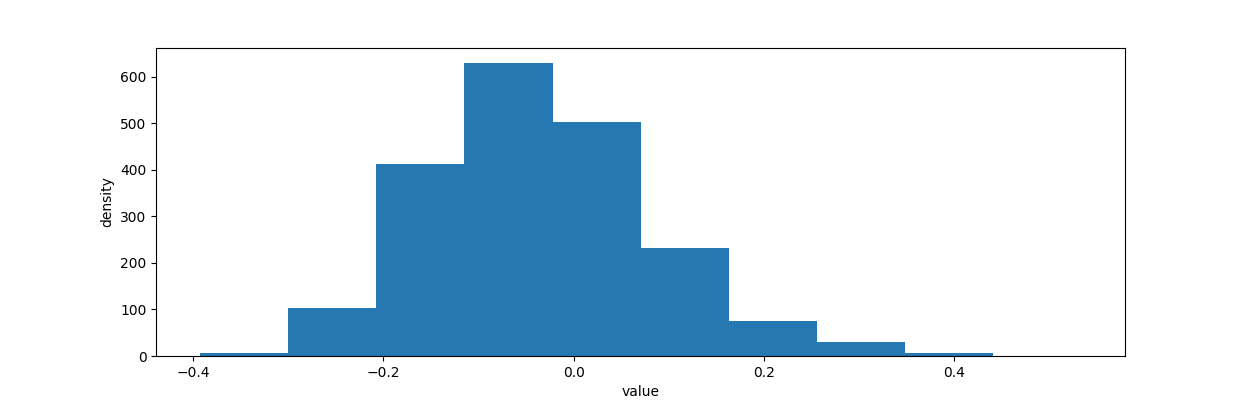1. 有89%的可能性，相对增幅会达到20%或者更多；
2. 有72%的可能性，增幅能达到50%；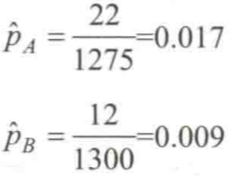### 3. 创建后验概率增幅的点估计

1. 返回增幅后验分布的均值：这个方法并不是非常好。原因在于对于一个倾斜的长尾分布，类似均值这样的统计量会很受影响，因而结论会过分表达长尾数据以至于高估实际的相对增幅；
2. 返回增幅后验分布的中位数：中位数应该是更合理的值，它对于倾斜的分布会更有鲁棒性。然而在实践中，中位数仍然可能导致结论被高估；
3. 返回增幅后验分布的百分位数（低于50%）。比如，返回第30%百分位数。这样做会有两个想要的特性：
1）它相当于从数学上在增幅后验分布之上应用了一个损失函数。以惩罚过高的估计，这样估计的结果就更加保守
2）随着我们得到越来越多的实验数据，增幅的后验分布会越来越窄，意味着任何百分位数都会收敛到同一个点

# -*- coding: utf-8 -*-

import numpy as np
import matplotlib.pyplot as plt
import scipy.stats as stats
import pymc as pm
from scipy.stats import beta
from IPython.core.pylabtools import figsize

visitors_to_A = 1275
visitors_to_B = 1300
conversions_from_A = 22
conversions_from_B = 12

alpha_prior = 1
beta_prior = 1

posterior_A = beta(alpha_prior + conversions_from_A,
beta_prior + visitors_to_A - conversions_from_A)

posterior_B = beta(alpha_prior + conversions_from_B,
beta_prior + visitors_to_B - conversions_from_B)

samples = 20000
samples_posterior_A = posterior_A.rvs(samples)
samples_posterior_B = posterior_B.rvs(samples)

print(samples_posterior_A > samples_posterior_B).mean()

def relative_increase(a, b):
return (a - b) / b

posterior_rel_increase = relative_increase(samples_posterior_A, samples_posterior_B)

mean = posterior_rel_increase.mean()
median = np.percentile(posterior_rel_increase, 50)
conservtive_percentile = np.percentile(posterior_rel_increase, 30)

figsize(12, 4)

plt.hist(posterior_rel_increase, label='relative increase')
plt.vlines(mean, 0, 2500, linestyles='-.', label='mean')
plt.vlines(median, 0, 2500, linestyles=':', label='median')
plt.vlines(conservtive_percentile, 0, 2500, linestyles='--', label='conservtive_percentile')
plt.xlabel('value')
plt.ylabel('density')
plt.show()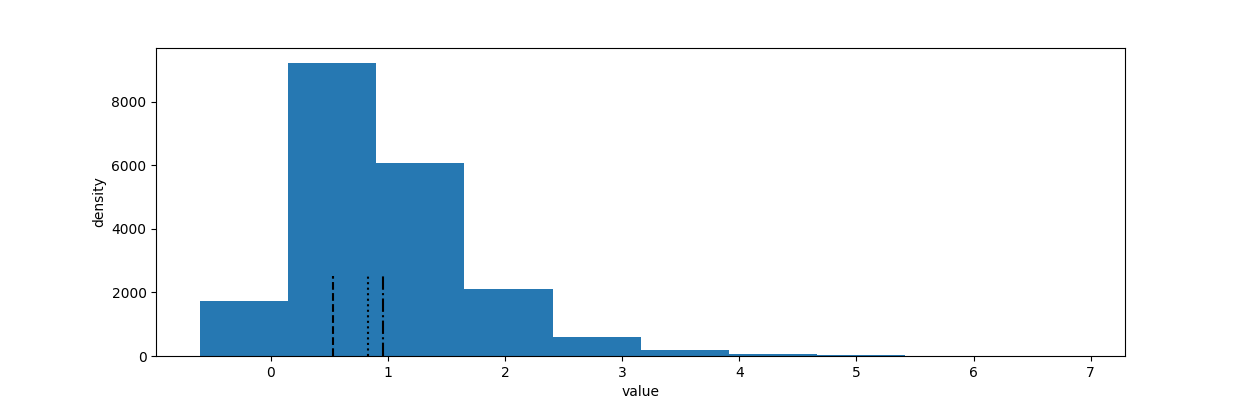posted @ 2019-02-27 22:15  郑瀚Andrew.Hann  阅读(...)  评论(... 编辑 收藏